Next: References and further reading Up: Variant tf-idf functions Previous: Document and query weighting   Contents   Index

## Pivoted normalized document length

In Section 6.3.1 we normalized each document vector by the Euclidean length of the vector, so that all document vectors turned into unit vectors. In doing so, we eliminated all information on the length of the original document; this masks some subtleties about longer documents. First, longer documents will - as a result of containing more terms - have higher tf values. Second, longer documents contain more distinct terms. These factors can conspire to raise the scores of longer documents, which (at least for some information needs) is unnatural. Longer documents can broadly be lumped into two categories: (1) verbose documents that essentially repeat the same content - in these, the length of the document does not alter the relative weights of different terms; (2) documents covering multiple different topics, in which the search terms probably match small segments of the document but not all of it - in this case, the relative weights of terms are quite different from a single short document that matches the query terms. Compensating for this phenomenon is a form of document length normalization that is independent of term and document frequencies. To this end, we introduce a form of normalizing the vector representations of documents in the collection, so that the resulting normalized'' documents are not necessarily of unit length. Then, when we compute the dot product score between a (unit) query vector and such a normalized document, the score is skewed to account for the effect of document length on relevance. This form of compensation for document length is known as pivoted document length normalization .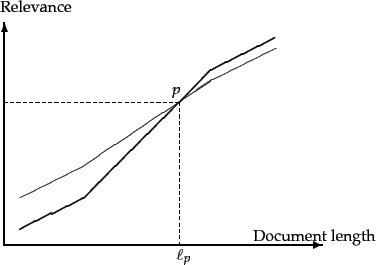Consider a document collection together with an ensemble of queries for that collection. Suppose that we were given, for each query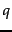and for each document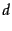, a Boolean judgment of whether or notis relevant to the query; in Chapter 8 we will see how to procure such a set of relevance judgments for a query ensemble and a document collection. Given this set of relevance judgments, we may compute a probability of relevance as a function of document length, averaged over all queries in the ensemble. The resulting plot may look like the curve drawn in thick lines in Figure 6.16 . To compute this curve, we bucket documents by length and compute the fraction of relevant documents in each bucket, then plot this fraction against the median document length of each bucket. (Thus even though the curve'' in Figure 6.16 appears to be continuous, it is in fact a histogram of discrete buckets of document length.)

On the other hand, the curve in thin lines shows what might happen with the same documents and query ensemble if we were to use relevance as prescribed by cosine normalization Equation 27 - thus, cosine normalization has a tendency to distort the computed relevance vis-à-vis the true relevance, at the expense of longer documents. The thin and thick curves crossover at a point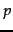corresponding to document length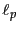, which we refer to as the pivot length; dashed lines mark this point on the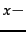and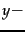axes. The idea of pivoted document length normalization would then be to rotate'' the cosine normalization curve counter-clockwise aboutso that it more closely matches thick line representing the relevance vs. document length curve. As mentioned at the beginning of this section, we do so by using in Equation 27 a normalization factor for each document vector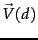that is not the Euclidean length of that vector, but instead one that is larger than the Euclidean length for documents of length less than, and smaller for longer documents.

To this end, we first note that the normalizing term forin the denominator of Equation 27 is its Euclidean length, denoted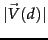. In the simplest implementation of pivoted document length normalization, we use a normalization factor in the denominator that is linear in, but one of slope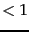as in Figure 6.17 . In this figure, theaxis represents, while theaxis represents possible normalization factors we can use. The thin line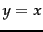depicts the use of cosine normalization. Notice the following aspects of the thick line representing pivoted length normalization:

1. It is linear in the document length and has the form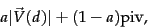(31)

where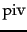is the cosine normalization value at which the two curves intersect.
2. Its slope is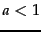and (3) it crosses theline at piv.
It has been argued that in practice, Equation 31 is well approximated by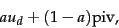(32)

where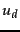is the number of unique terms in document.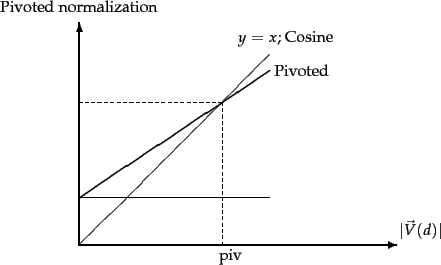Of course, pivoted document length normalization is not appropriate for all applications. For instance, in a collection of answers to frequently asked questions (say, at a customer service website), relevance may have little to do with document length. In other cases the dependency may be more complex than can be accounted for by a simple linear pivoted normalization. In such cases, document length can be used as a feature in the machine learning based scoring approach of Section 6.1.2 .

Exercises.

• One measure of the similarity of two vectors is the Euclidean distance (or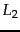distance ) between them: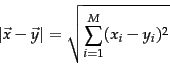(33)

Given a queryand documents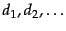, we may rank the documents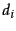in order of increasing Euclidean distance from. Show that ifand theare all normalized to unit vectors, then the rank ordering produced by Euclidean distance is identical to that produced by cosine similarities.

• Compute the vector space similarity between the query digital cameras'' and the document digital cameras and video cameras'' by filling out the empty columns in Table 6.1 . Assume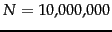, logarithmic term weighting (wf columns) for query and document, idf weighting for the query only and cosine normalization for the document only. Treat and as a stop word. Enter term counts in the tf columns. What is the final similarity score?

Table 6.1: Cosine computation for Exercise 6.4.4 .
 query document word tf wf df idf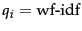tf wf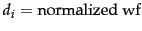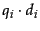digital 10,000 video 100,000 cameras 50,000

• Show that for the query affection, the relative ordering of the scores of the three documents in Figure 6.13 is the reverse of the ordering of the scores for the query jealous gossip.

• In turning a query into a unit vector in Figure 6.13, we assigned equal weights to each of the query terms. What other principled approaches are plausible?

• Consider the case of a query term that is not in the set of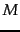indexed terms; thus our standard construction of the query vector results in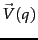not being in the vector space created from the collection. How would one adapt the vector space representation to handle this case?

• Refer to the tf and idf values for four terms and three documents in Exercise 6.2.2. Compute the two top scoring documents on the query best car insurance for each of the following weighing schemes: (i) nnn.atc; (ii) ntc.atc.

• Suppose that the word coyote does not occur in the collection used in Exercises 6.2.2 and 6.4.4. How would one compute ntc.atc scores for the query coyote insurance?Next: References and further reading Up: Variant tf-idf functions Previous: Document and query weighting   Contents   Index